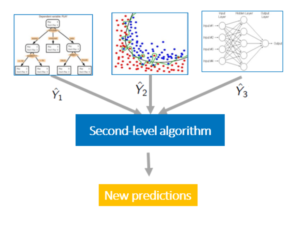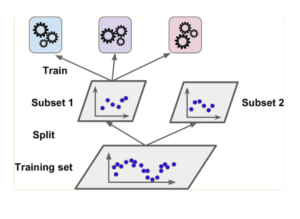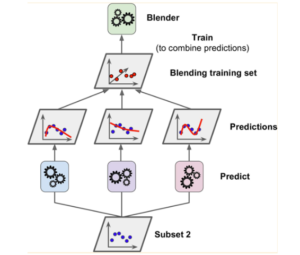Blending Algorithms in Machine LearningMl wizard

The Ensemble technique is one of the best-performing techniques used in the field of machine learning for getting better results on complex types of datasets. There are many ensemble techniques available that use multiple machine-learning algorithms for training on the same dataset and return great results compared to the single normal machine-learning algorithms.

In this article we will be discussing the blending algorithms with its core intuition behind other working mechanisms and the data sampling styles n it with the code examples, concluding with some key takeaways to remember. Knowledge about these techniques will help one to understand the power of the ensemble techniques better and also it will help one to answer the question related to blending efficiently.

# Table of Content

1. Blending: How it Works
2. Problem with Blending
3. Hold Out Approach: Blending
4. Blending: Code Example
5. Stacking vs Blending
6. Conclusion

# Blending: How it works

As we know that blending is the ensemble technique, it uses multiple machine learning algorithms for training on the same dataset. Here unlike other ensemble techniques, there are two layers of machine-learning algorithms are used, one is base models and the second layer algorithms are known as meta models.

the base models are trained on the training dataset provided first, once the base models are trained on the dataset, the test set is fed to the base models for prediction, and the predicted values from the base models are used for the training of the meta-model. and the meta-models are trained on the prediction data, after training the meta-model assigns the weights to the base models and the output from the meta-models Is considered the final output.Image Source

For example, let’s suppose we have 3 machine learning algorithms as base models and 1 algorithm as the meat model, so in the first step, the base model will be trained on the same dataset. Once trained, the test data is fed to all three base models and all the base models will predict different values for t same test data. Now, these predictions from all the individual base models will be used for training the meta-model, once the meta-model is trained, no the output from the meta-models will be served as the final output.

# Problem with Blending

As we know that blending algorithms consist of base and meta models, the training of these algorithms is done on the same dataset, so here first the base models are trained on the same dataset and the outputs from the base models are again used as the training set for metamodels, so here there is a problem of data leakage associated where the overfitting can occur and the performance of the model on training data will be very well but on testing data, the model will perform very poor.

Here, in this case, to tackle the problem of overfitting, the hold-out approach is used of tracking this problem. This approach is also known as blending.

# Hold Out Approach: Blending

The hold-out approach is used to tackle the problems of overfitting where due to the date leakage the model will perform poorly on the unknown or the testing dataset. here in this approach in the first step, the main dataset is divided into training and testing sets, which can be easily done by the train_test_split module.Image Source

Once the data is split into the training and testing set, in step 2, again the training set is divided into two parts which are the training of the base models set and the validation set.Image Source

Once all the splitting on the dataset is done, the base models are trained on the training base models set, once trained the validation data is fed to the base models on which they will return prediction data. Now the resulting data will be used as the training data of the meta-models, where the meta-model will be trained and the results from the meta-models will be considered as the final output of the model. The test set which is obtained in the first step can be used to evaluate the blending model.

# Blending: Code Example

To apply the blending algorithm, one will need to write the code manually, as there is not any built-in module created for this algorithm.

To apply blending on any dataset, use the code below:

Importing Required Libraries:

``````from numpy import hstack
from sklearn.datasets import make_classification
from sklearn.model_selection import train_test_split
from sklearn.metrics import accuracy_score
from sklearn.linear_model import LogisticRegression
from sklearn.neighbors import KNeighborsClassifier
from sklearn.tree import DecisionTreeClassifier
from sklearn.svm import SVC
from sklearn.naive_bayes import GaussianNB

``````

Creating base Models List:

``````def get_models():
models = list()
models.append(('lr', LogisticRegression()))
models.append(('knn', KNeighborsClassifier()))
models.append(('cart', DecisionTreeClassifier()))
models.append(('svm', SVC()))
models.append(('bayes', GaussianNB()))
return models

``````

Fit the ensembles:

``````def fit_ensemble(models, X_train, X_val, y_train, y_val):
meta_X = list()
for name, model in models:
# fit in training set
model.fit(X_train, y_train)
# predict on hold out set
yhat = model.predict(X_val)
# reshape predictions into a matrix with one column
yhat = yhat.reshape(len(yhat), 1)
# store predictions as input for blending
meta_X.append(yhat)
# create 2d array from predictions, each set is an input feature
meta_X = hstack(meta_X)
# define blending model
blender = LogisticRegression()
# fit on predictions from base models
blender.fit(meta_X, y_val)
return blender
def predict_ensemble(models, blender, X_test):
# make predictions with base models
meta_X = list()
for name, model in models:
# predict with base model
yhat = model.predict(X_test)

yhat = yhat.reshape(len(yhat), 1)
# store prediction
meta_X.append(yhat)
# create 2d array from predictions, each set is an input feature
meta_X = hstack(meta_X)
# predict
return blender.predict(meta_X)
# create the base models
models = get_models()
# train the blending ensemble
blender = fit_ensemble(models, X_train, X_val, y_train, y_val)
# make predictions on test set
yhat = predict_ensemble(models, blender, X_test)
# evaluate predictions
score = accuracy_score(y_test, yhat)
print('Blending Accuracy: %.3f' % (score*100))

``````

# Stacking vs Blending

As stacking and blending are similar ensemble techniques, the question might come to our mind, what is the difference between them, and which is a better approach?

Well, the first basic difference between these two approaches is the sampling of the data, In stacking the K Fold approach is used where using the value of K, the dataset is split, whereas in blending the dataset is normally split into two times and the validation data is achieved.

The second very intuitive difference is that in stacking the meta-models are trained first and then the base models are trained whereas in blending the base models are trained first and then the meta-models are trained.

# Conclusion

In this article, the blending ensemble technique is discussed with its core intuition, working mechanisms, examples, and the code to develop the blender. Referring to this article will help one to better understand the blending and clear the concepts related to it in a better way. This article will also help one to answer the questions related to blending in a very efficient way.